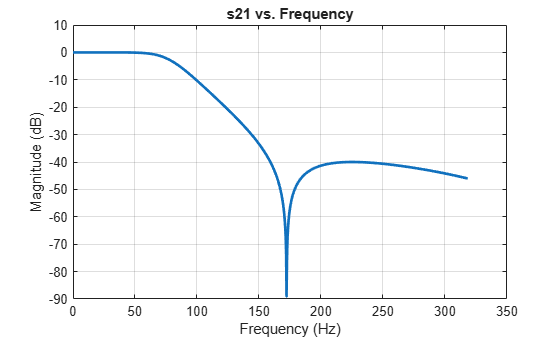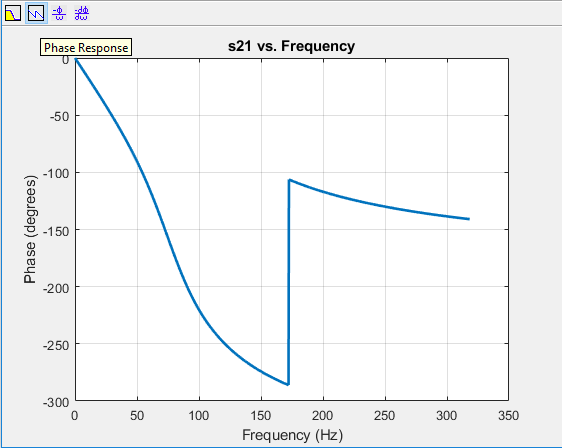Main Content

# Design, visualize and explore Inverse Chebyshev filter - II

This example shows how to design a fourth-order inverse Chebyshev low-pass filter with stopband frequency of 10000 rad/sec, and epsilon of 0.01 (please see the reference section) using `rffilter`. This `rffilter` could be used in a `circuit` or in a `rfbudget` object.

The `rffilter` object is used to design a RF filter. A filter requires a minimum set for parameters to completely define it.

The parameters to design an inverse Chebyshev filter can be one of the following:

• Filter order, Passband frequency, Passband and Stopband Attenuation

• Passband and Stopband frequencies, Passband and Stopband Attenuation

• Filter order, Stopband frequency, Stopband Attenuation

### Design Filter

```N = 4; % Filter order Fs = 1000/(2*pi); % Stopband frequency epsilon = 0.01; Rs = 10*log10((1+epsilon^2)/epsilon^2); % Stopband attenuation```

Use the first set of parameters to define the filter.

```r = rffilter('FilterType','InverseChebyshev','ResponseType','Lowpass', ... 'Implementation','Transfer function','FilterOrder',N, ... 'PassbandFrequency',Fs,'PassbandAttenuation',Rs, ... 'StopbandAttenuation',Rs);```

Note: Alternative, you can also use the third set of parameters to design the same filter:

```r = rffilter('FilterType','InverseChebyshev','ResponseType','Lowpass', ... 'Implementation','Transfer function','FilterOrder',N, ... 'StopbandFrequency',Fs,'StopbandAttenuation',Rs); ```

The limitation of this parameter set is that it assumes the passband attenuation to be fixed at 10*log10(2) dB.

### Visualize magnitude response, phase response, and phase delay of filter

```frequencies = linspace(0,2*Fs,1001); rfplot(r, frequencies);```Optionally, you can also use Signal Processing Toolbox to visualize the analog filter using:

```freqs(numerator{2,1},denominator) ```

### Find zeros, poles, and gain

`[z,p,k] = zpk(r);`

You can obtain zeros, poles, and gain of Transfer function (S21) by:

```format long g zeros_21 = z{2,1}```
```zeros_21 = 4×1 complex 0 + 1082.39220029239i 0 - 1082.39220029239i 0 + 2613.12592975275i 0 - 2613.12592975275i ```
`poles_21 = p % Same denominator for S11, S12, S21 and S22`
```poles_21 = 4×1 complex -171.158733950657 + 476.096694464131i -171.158733950657 - 476.096694464131i -504.530434776367 + 240.786480832184i -504.530434776367 - 240.786480832184i ```
`k_21 = k{2,1}`
```k_21 = 0.00999950003749688 ```

### View transfer function in factorized form

View these factor forms directly from the filter r.

`disp('Numerator of Transfer function as factors:');`
```Numerator of Transfer function as factors: ```
`r.DesignData.Numerator21`
```ans = 2×3 1 0 1171572.87525381 0.00999950003749688 0 68280.8572899443 ```
`disp('Denominator of Transfer function as factors:');`
```Denominator of Transfer function as factors: ```
`r.DesignData.Denominator`
```ans = 2×3 1 342.317467901314 255963.374687264 1 1009.06086955273 312529.088967178 ```

Alternatively, use |zpk| from Control System Toolbox to view the transfer function in factorized form.

`G_s = zpk(zeros_21,poles_21,k_21)`
```G_s = 0.0099995 (s^2 + 1.172e06) (s^2 + 6.828e06) ------------------------------------------------- (s^2 + 1009s + 3.125e05) (s^2 + 342.3s + 2.56e05) Continuous-time zero/pole/gain model. ```

 Paarmann, L. D. Design and Analysis of Analog Filters: A Signal Processing Perspective. SECS 617. Boston: Kluwer Academic Publishers, 2001.

Download ebook